NCERT Exemplar - MCQs

Chapter 6 Class 12 Application of Derivatives
Serial order wise

## (C) x + 3y ± 8 = 0         (D) x + 3y = 0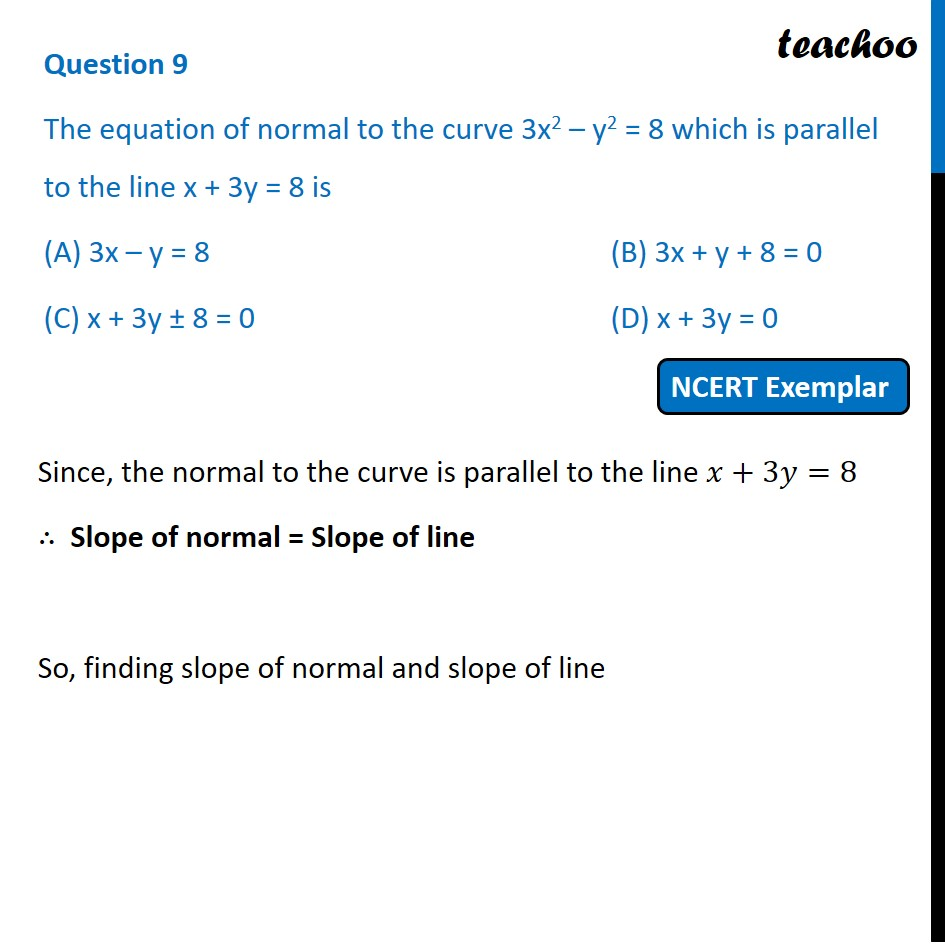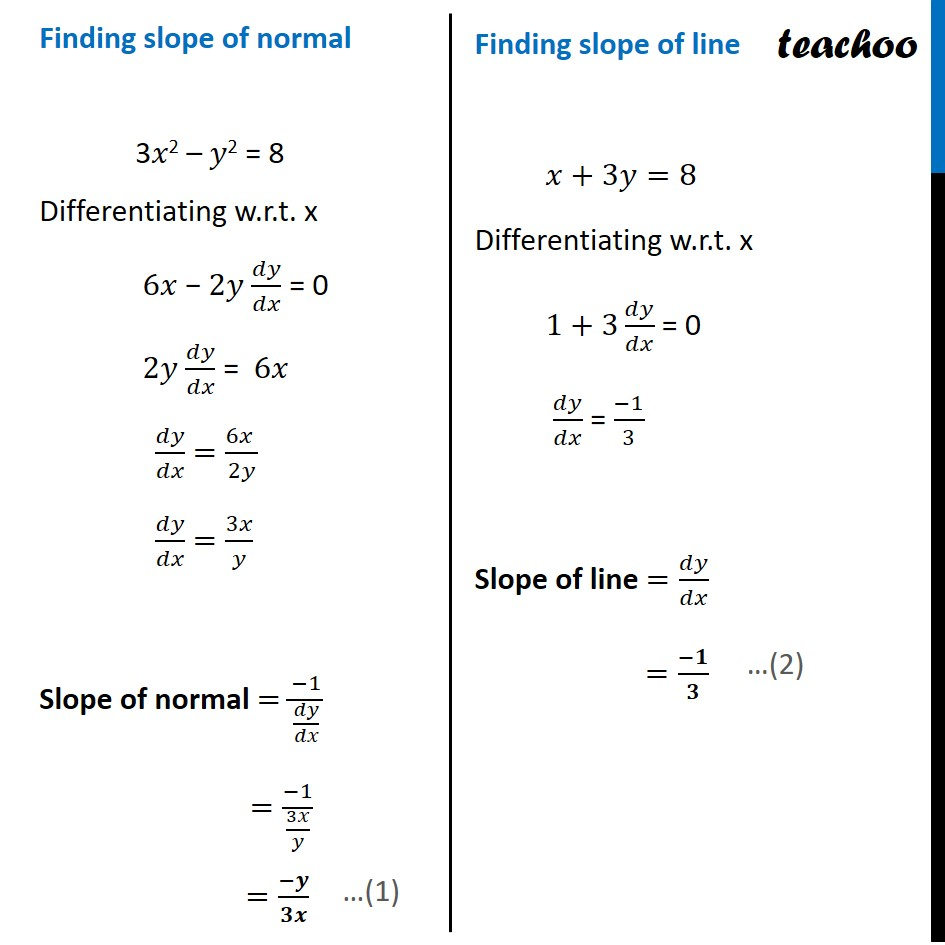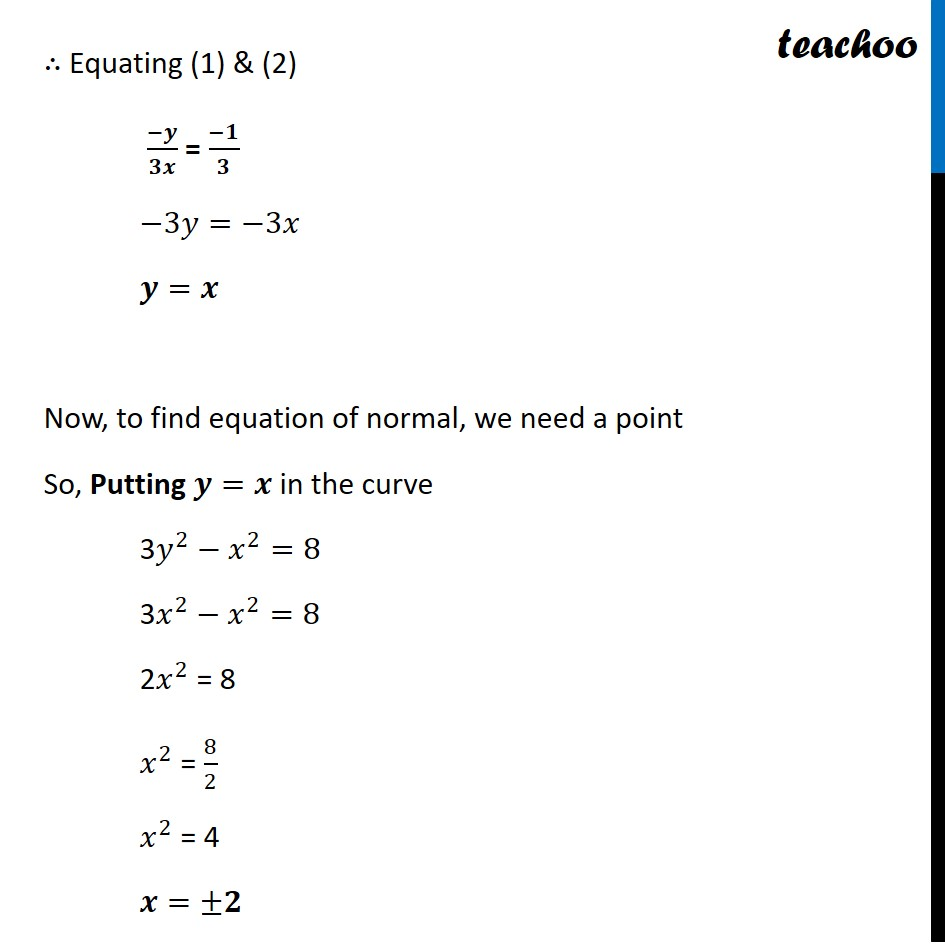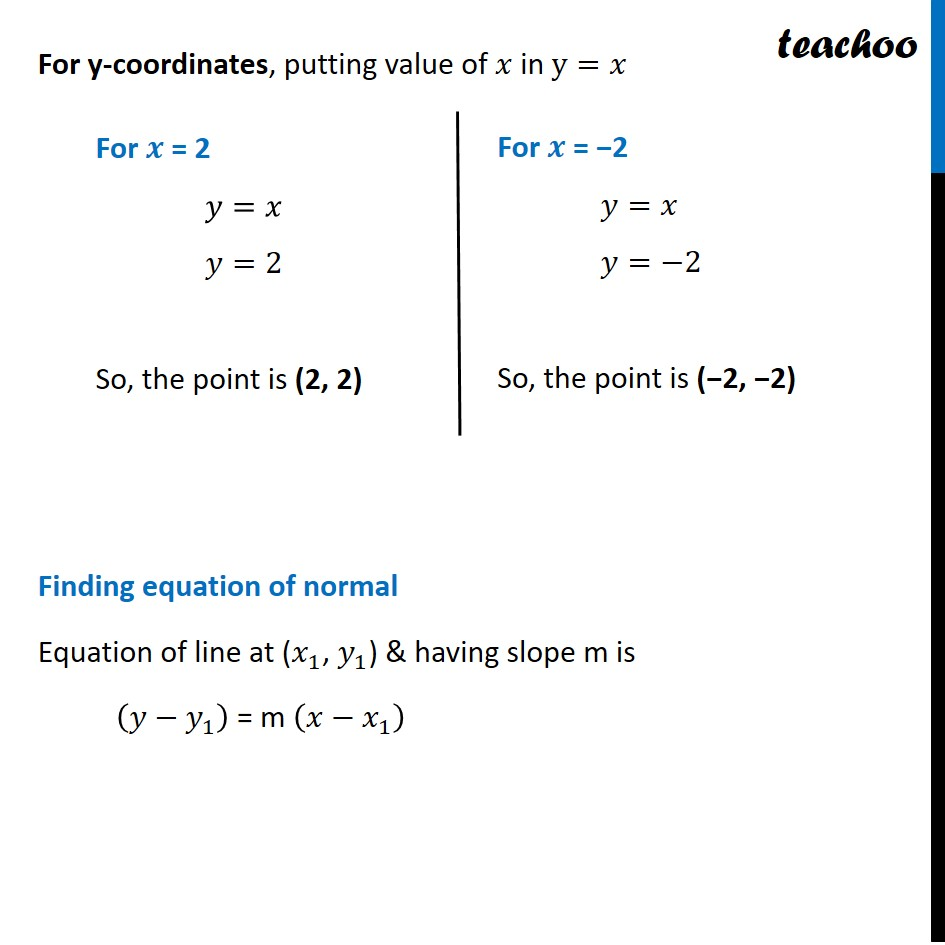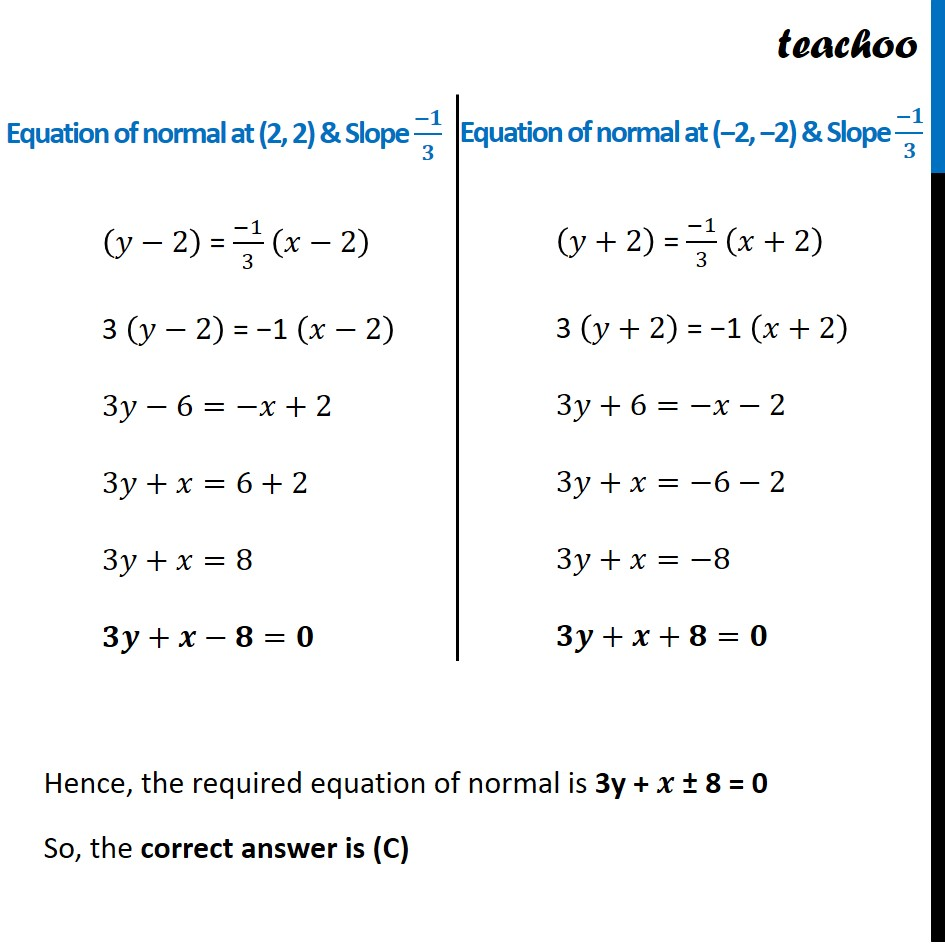Learn in your speed, with individual attention - Teachoo Maths 1-on-1 Class

### Transcript

Question 7 The equation of normal to the curve 3x2 – y2 = 8 which is parallel to the line x + 3y = 8 is (A) 3x – y = 8 (B) 3x + y + 8 = 0 (C) x + 3y ± 8 = 0 (D) x + 3y = 0 Since, the normal to the curve is parallel to the line 𝑥+3𝑦=8 ∴ Slope of normal = Slope of line So, finding slope of normal and slope of line Finding slope of normal "3" 𝑥"2 –" 𝑦"2 = 8" Differentiating w.r.t. x 6𝑥 − 2𝑦 𝑑𝑦/𝑑𝑥 = 0 2𝑦 𝑑𝑦/𝑑𝑥 = 6𝑥 𝑑𝑦/𝑑𝑥 =(6𝑥 )/2𝑦 𝑑𝑦/𝑑𝑥 =3𝑥/𝑦 Slope of normal =(−1)/(𝑑𝑦/𝑑𝑥) =(−1)/(3𝑥/𝑦) =(−𝒚)/𝟑𝒙 Finding slope of line 𝑥+3𝑦=8 Differentiating w.r.t. x 1+3 𝑑𝑦/𝑑𝑥 = 0 𝑑𝑦/𝑑𝑥 = (−1)/3 Slope of line =𝑑𝑦/𝑑𝑥 =(−𝟏)/𝟑 ∴ Equating (1) & (2) (−𝒚)/𝟑𝒙 = (−𝟏)/𝟑 −3𝑦=−3𝑥 𝒚=𝒙 Now, to find equation of normal, we need a point So, Putting 𝒚=𝒙 in the curve 3𝑦^2−𝑥^2=8 3𝑥^2−𝑥^2=8 2𝑥^2 = 8 𝑥^2 = 8/2 𝑥^2 = 4 𝒙=±𝟐 For y-coordinates, putting value of 𝑥 in y=𝑥 Finding equation of normal Equation of line at (𝑥_1, 𝑦_1) & having slope m is (𝑦−𝑦_1 ) = m (𝑥−𝑥_1 ) For 𝒙 = 2 𝑦=𝑥 𝑦=2 So, the point is (2, 2) For 𝒙 = −2 𝑦=𝑥 𝑦=−2 So, the point is (−2, −2) Equation of normal at (2, 2) & Slope (−𝟏)/𝟑 (𝑦−2) = (−1)/3 (𝑥−2) 3 (𝑦−2) = −1 (𝑥−2) 3𝑦−6=−𝑥+2 3𝑦+𝑥=6+2 3𝑦+𝑥=8 𝟑𝒚+𝒙−𝟖=𝟎 Equation of normal at (−2, −2) & Slope (−𝟏)/𝟑 (𝑦+2) = (−1)/3 (𝑥+2) 3 (𝑦+2) = −1 (𝑥+2) 3𝑦+6=−𝑥−2 3𝑦+𝑥=−6−2 3𝑦+𝑥=−8 𝟑𝒚+𝒙+𝟖=𝟎 Hence, the required equation of normal is 3y + 𝒙 ± 8 = 0 So, the correct answer is (C)